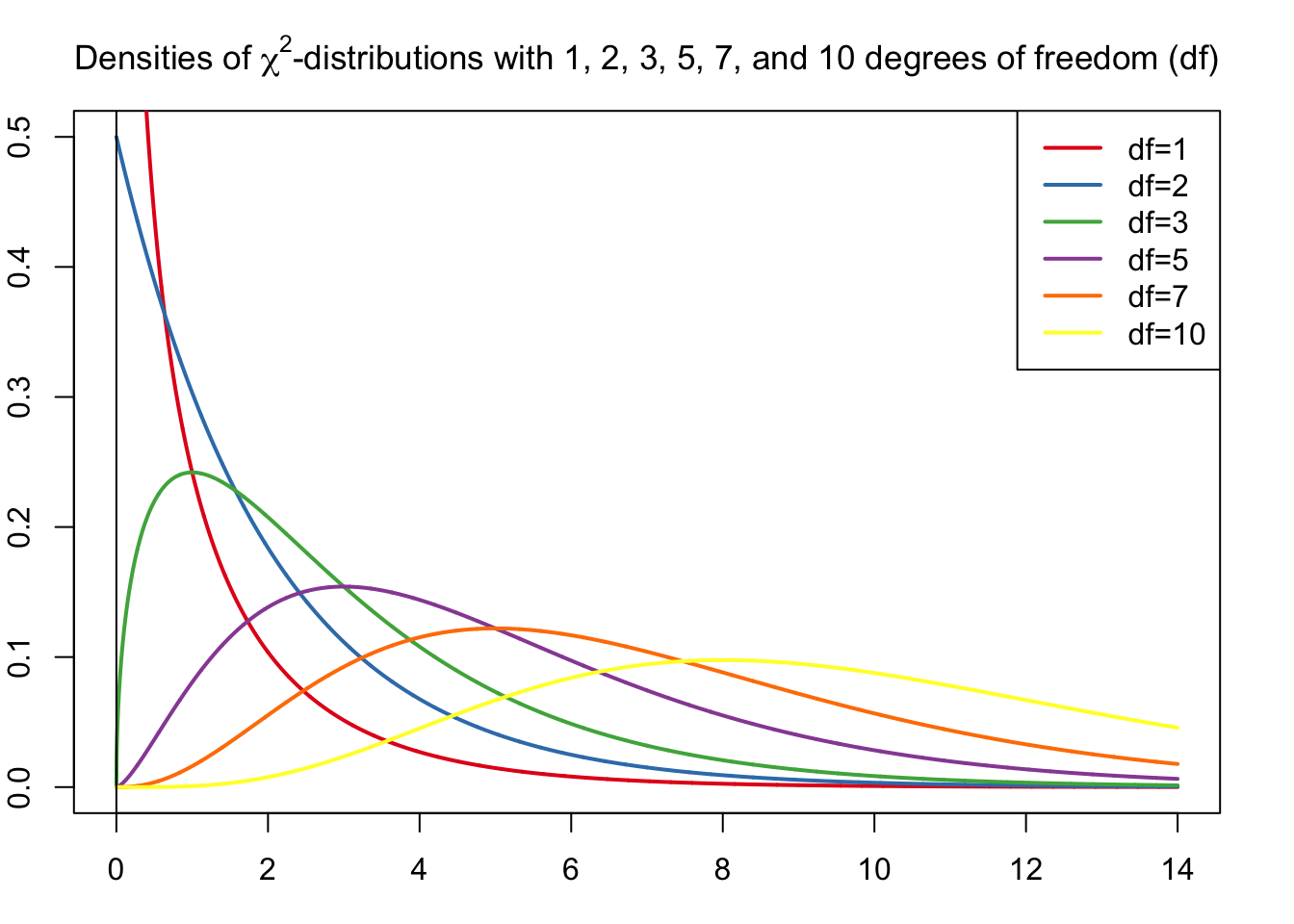In addition to inferential methods for hypothesis tests for population parameters, such as the mean, $$\mu$$, and the standard deviation, $$\sigma$$, there are statistical methods to make inferences about the distribution of a variable. These inferential procedures rely on the chi-square ($$\chi^2$$) distribution, and thus are called $$\chi^2$$-tests.

In the following section we discuss the chi-square goodness-of-fit test, a hypothesis test that is applied to make inferences about the distribution of a variable and the chi-square independence test, a hypothesis test that is applied to decide whether an association exists between two variables of a population.

#### $$\chi^2$$-Distribution

Basic Properties of $$\chi^2$$-Curves (Weiss 2010)

• The total area under a $$\chi^2$$-curve equals 1.
• A $$\chi^2$$-curve starts at 0 on the horizontal axis and extends indefinitely to the right, approaching, but never touching, the horizontal axis as it does so.
• A $$\chi^2$$-curve is right skewed.
• As the number of degrees of freedom becomes larger, $$\chi^2$$- curves look increasingly like normal curves.